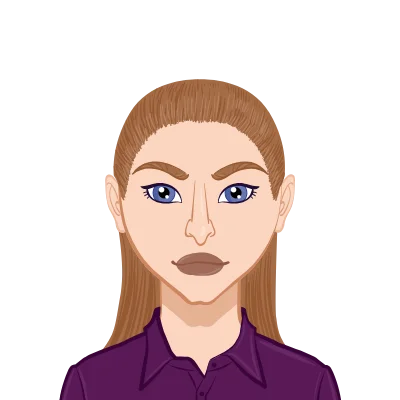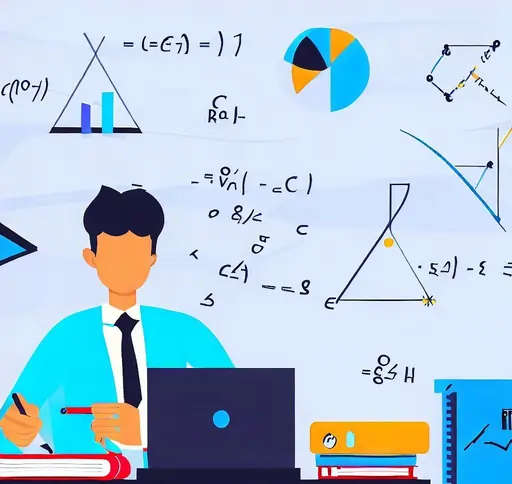# Quantum Algorithms: A Mathematical Introduction

September 07, 2023Cordelia Matheson
United States of America
Quantum Algorithms
Cordelia Matheson is a distinguished scholar in the realm of Quantum Algorithms and Quantum Computing, known for her groundbreaking research and insightful contributions to the field.
Quantum computing is a field that has garnered significant attention in recent years, promising to revolutionize the world of computation. At the heart of this quantum revolution are quantum algorithms—powerful problem-solving techniques designed to harness the unique properties of quantum mechanics. In this blog, we will embark on a mathematical journey to introduce the fundamental concepts behind quantum algorithms, all without delving into complex calculations and formulas. Successfully completing your Quantum Algorithm assignment requires a deep grasp of quantum computing principles and their skillful application.

## What Makes Quantum Computers Special?

Before delving into quantum algorithms, it's essential to understand why quantum computers have the potential to outperform classical computers in certain tasks. Traditional computers rely on bits, which can represent either a 0 or a 1. Quantum computers, on the other hand, use quantum bits or qubits. Unlike classical bits, qubits can exist in a superposition of both 0 and 1 states simultaneously, thanks to the principles of quantum mechanics. This property allows quantum computers to process vast amounts of information in parallel, potentially solving complex problems much faster than classical computers.### Quantum Gates: The Building Blocks of Quantum Computation

Quantum gates are the fundamental building blocks of quantum computation, serving a role analogous to classical logic gates in conventional computing. While classical computers manipulate bits using operations like AND, OR, and NOT gates, quantum computers operate on qubits, the quantum analog of classical bits. These qubits can exist in a superposition of states, allowing quantum gates to perform a wide range of operations simultaneously. Here, we'll delve into some of the essential quantum gates and their functions:

2. The Hadamard gate is one of the most fundamental quantum gates. It's often the starting point for many quantum algorithms because it plays a pivotal role in creating superpositions. When you apply the Hadamard gate to a qubit initially in the state |0⟩ (representing classical bit 0), it transforms it into the superposition state (|0⟩ + |1⟩) / √2. Similarly, if applied to |1⟩, it becomes (|0⟩ - |1⟩) / √2. In essence, the Hadamard gate places the qubit into a balanced superposition of its basis states, making it a versatile tool for quantum computations.

3. Pauli-X Gate (X):
4. The Pauli-X gate is the quantum equivalent of the classical NOT gate. When applied to a qubit, it flips its state. So, if you apply the Pauli-X gate to |0⟩, it becomes |1⟩, and vice versa. This gate is particularly useful for changing the state of a qubit, a fundamental operation in many quantum algorithms.

5. Pauli-Y Gate (Y) and Pauli-Z Gate (Z):
6. The Pauli-Y and Pauli-Z gates are responsible for rotations around different axes in the Bloch sphere representation of qubits. The Pauli-Y gate rotates the qubit's state about the Y-axis, creating a combination of |0⟩ and |1⟩ states along with complex phase differences. The Pauli-Z gate, on the other hand, rotates the qubit's state about the Z-axis, introducing a phase shift without changing the probabilities of measuring |0⟩ or |1⟩. These gates are crucial for more advanced quantum computations and quantum error correction.

7. CNOT (Controlled-NOT) Gate:
8. The CNOT gate is a two-qubit gate, and it's the foundation of quantum entanglement, a unique quantum phenomenon. This gate operates conditionally, meaning it performs an operation on the second qubit based on the state of the first qubit. If the control qubit (the first one) is in state |1⟩, the CNOT gate flips the target qubit's state. However, if the control qubit is |0⟩, it leaves the target qubit unchanged. The CNOT gate's ability to create entanglement between qubits and perform conditional operations is essential in many quantum algorithms, including quantum teleportation and quantum error correction.

## Quantum Circuit Design

Quantum gates are not used in isolation but are combined in quantum circuits to perform complex computations. Quantum circuits are constructed by arranging gates in a specific sequence, often designed with meticulous care to optimize the solution to a particular problem. These circuits take advantage of quantum properties like superposition and entanglement to explore multiple possibilities simultaneously.

Quantum gates are the heart of quantum computation, enabling the manipulation of qubits in ways that classical logic gates cannot achieve. By applying gates like the Hadamard gate, Pauli-X, Pauli-Y, Pauli-Z, and the CNOT gate, quantum algorithms harness the power of quantum mechanics to solve problems more efficiently than classical counterparts. As quantum technology continues to advance, understanding and mastering these gates will become increasingly crucial for harnessing the full potential of quantum computing.

### Quantum Superposition: Exploring Multiple States Simultaneously

Quantum superposition is one of the foundational principles of quantum mechanics that distinguishes quantum systems from classical ones. It allows qubits to exist in a combination of multiple states simultaneously. In classical computing, a bit can only be in one of two states: 0 or 1. However, qubits can be in a superposition of these states, represented mathematically as a linear combination.

Imagine a classical coin. It can be in one of two states: heads or tails. In contrast, a quantum coin, or qubit, can be in a superposition of both heads and tails simultaneously. Mathematically, if |0⟩ and |1⟩ represent the basis states of a qubit, a superposition can be expressed as:

ψ = α|0⟩ + β|1⟩

Here, α and β are complex amplitudes that determine the probability of measuring the qubit in either state |0⟩ or |1⟩. The absolute squares of these amplitudes |α|² and |β|² give the probabilities of observing each outcome when measured. Importantly, |α|² + |β|² = 1, ensuring that the qubit's total probability is conserved.

## Superposition in Quantum Algorithms

Superposition is a powerful resource in quantum algorithms. It allows quantum computers to explore multiple solutions to a problem simultaneously. This property is leveraged in algorithms like Grover's algorithm, which can search an unsorted database much faster than classical counterparts by evaluating multiple database entries in parallel.

For instance, if you're searching for a specific item in an unsorted list, a classical computer would need to examine each item one by one. In contrast, Grover's algorithm uses superposition to simultaneously evaluate multiple items, significantly reducing the time required to find the desired item.

### Entanglement: Spooky Action at a Distance

Entanglement is another extraordinary phenomenon in quantum mechanics. It occurs when two or more qubits become correlated or intertwined in such a way that the state of one qubit is dependent on the state of another, even when they are separated by vast distances. This seemingly "spooky action at a distance," as Einstein famously referred to it, is one of the most intriguing aspects of quantum physics.

## The EPR Paradox and Entanglement

Entanglement was first highlighted in the famous EPR (Einstein-Podolsky-Rosen) paradox, a thought experiment devised by Einstein, Podolsky, and Rosen in 1935. In their scenario, two entangled particles, such as electrons, are created in such a way that measuring one particle's state instantaneously determines the state of the other, regardless of the distance separating them.

Entanglement defies classical intuition. It implies that information can be transmitted faster than the speed of light, which contradicts Einstein's theory of relativity. However, entanglement cannot be used to transmit information faster than the speed of light, as it doesn't allow for direct communication.

## Entanglement in Quantum Algorithms

Entanglement is a crucial resource in quantum algorithms, particularly in those that involve multiple qubits. When qubits are entangled, they share a highly correlated quantum state, which can be exploited to perform computations that classical computers struggle with.

Quantum teleportation is an example of how entanglement is used in quantum algorithms. It allows the transmission of the state of one qubit to another qubit, even when they are physically separated. This process relies on the entanglement between two qubits and classical communication to complete the teleportation.

Another application of entanglement is in quantum error correction, where entangled qubits can be used to detect and correct errors that naturally occur in quantum computations.

## The Quantum Oracle

In many quantum algorithms, a quantum oracle serves as the problem-specific component. This oracle is like a "black box" that implements a specific function. The famous example is Grover's algorithm, which searches an unsorted database. The quantum oracle in Grover's algorithm marks the solution to the search problem, making it a critical part of the algorithm.

### Quantum Algorithms: An Overview

Now, let's explore some fundamental quantum algorithms without delving too deep into the math.

1. Grover's Algorithm
2. Grover's algorithm is renowned for its ability to perform unstructured search quadratically faster than classical algorithms. Given an unsorted database with N entries, Grover's algorithm can find the correct item in approximately √N steps. This efficiency is especially significant when dealing with large datasets.

3. Shor's Algorithm
4. Shor's algorithm is a game-changer for cryptography. It efficiently factors large numbers into their prime components, a task that forms the basis of many encryption schemes. Shor's algorithm threatens the security of current public-key cryptography systems, such as RSA, by efficiently breaking them using quantum computers.

5. Quantum Fourier Transform
6. The Quantum Fourier Transform is the quantum analog of the classical Fourier Transform. It's a fundamental component of many quantum algorithms, including Shor's algorithm. The QFT allows quantum computers to efficiently perform certain types of computations, particularly those involving periodicity.

7. Deutsch-Jozsa Algorithm
8. The Deutsch-Jozsa algorithm is a simple yet illustrative quantum algorithm. It solves a problem related to determining whether a given function is constant or balanced. While its practical applications may be limited, it demonstrates the power of quantum computing by solving the problem in a single query, compared to the classical counterpart, which requires multiple queries.

## Quantum Algorithms in Practice

While these quantum algorithms showcase the potential of quantum computing, it's crucial to understand that building practical, scalable quantum computers and algorithms is still an ongoing challenge. Quantum computers currently available are in their infancy, and they face issues like decoherence, error rates, and limited qubit counts.

Nonetheless, researchers and companies are making remarkable progress. Quantum computing has the potential to revolutionize various fields, from cryptography to drug discovery, optimization, and beyond.

## Conclusion

Quantum algorithms are the key to unlocking the true potential of quantum computing. While they may seem daunting with their mathematical intricacies, their underlying concepts, like superposition, entanglement, and quantum gates, form the foundation of this exciting field. As quantum computers continue to evolve, we can anticipate groundbreaking advances in science, technology, and computation. Quantum algorithms are at the forefront of this revolution, promising a future where problems that once seemed insurmountable may become solvable in the blink of a quantum eye.